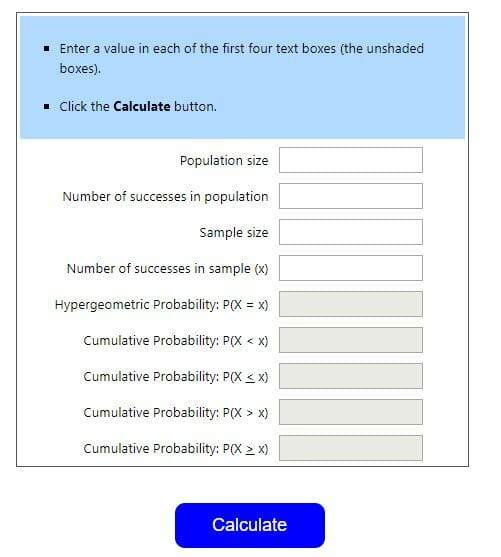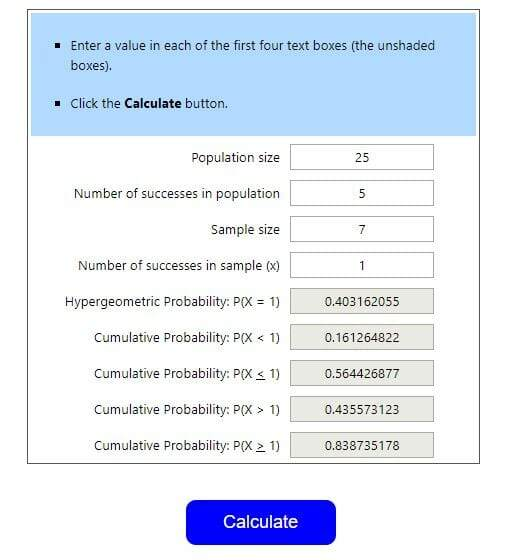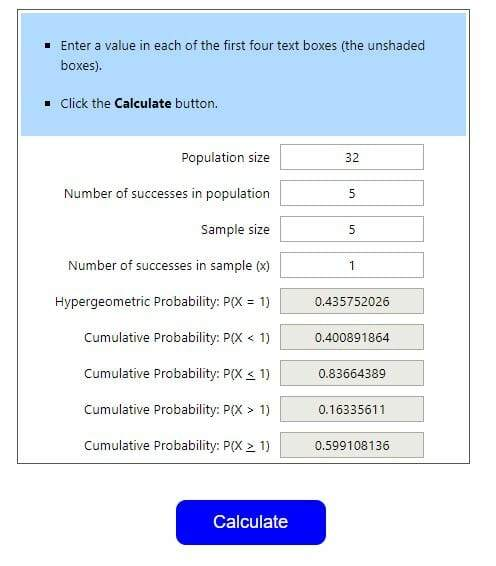Select Page

## Spoiler alert: game design uses math, whether you’re good at it or not.

Call it ‘math’ or ‘maths’ if you like – whatever you call it, I’ve never been a fan of it. I think it was around the time when letters started replacing numbers and I lost the ability to think as abstractly as the math classes required. I was a Business major in college, and did fine enough in the math classes I was required to take. They weren’t classes I was exactly thrilled by, but I got through them in time. albeit with much more effort than other classes.

Designing a game means one is working with probabilities, and changing them to fit your needs is part of the design process. You’ll also want to know how often (or rarely) a certain thing may happen, Designing a game means thinking about permutations, combinations, and might even involve a hypergeometric calculator. (Yes, this is a real thing. It’s pretty cool).

## The… hypergeometric calculator?!?

Yep, that’s what it’s called. It’s a really fancy way of saying ‘the probability of something happening’.  If there are five bad cards in a deck of 25 cards, what are the chances of drawing, say, 1 bad card when you draw 7 cards?  I could play hundreds of rounds and count how often this oddity happened, but math saves plenty of time and effort.

Start by going to http://stattrek.com/online-calculator/hypergeometric.aspx:Stat Trek asks for four things here, so let’s plug-in some numbers from my previous example (From my previous example (“If there are 5 bad cards in a deck of 25 cards, what are the chances of drawing, say, 1 bad card when you draw 7 cards?”)

• Population size is the overall universe or deck of cards to choose from. Here, it’s 25.
• Number of Successes in Population is the number of bad cards, or the cards you’re trying to see the probability. Here, it’s 5.
• Sample Size is the number of cards you’re drawing from that universe or deck of cards. Here, it’s 7.
• Number of successes in sample (x) is the number of bad cards, or the cards you’re trying to see the probabiliity in that sample size. Here, it’s 1.

Type all those into the calculator, and….OK, so the grey boxes got filled in. How does this help me? The grey boxes show the probabilities of that thing happening from 0 to 1, or 0% to 100%. Multiply each number by 100 to get their percentage.

That first box, P(X = 1)? That’s the one you want. Given this set up, the probability that you’ll draw exactly 1 bad card when you draw 7 cards is 0.403162055, or 40.3%. The other numbers are cumulative probabilities, which consider all the other options:

• Cumulative Probability: P(X < 1): 0.161264822 — the chance you’ll draw less than 1 bad card (in other words, 0) is 16.1%.
• Cumulative Probability: P(X < 1) : 0.564426877 — the chance you’ll draw 1 or fewer bad cards (in other words, 0 or 1) is 56.4%.
• Cumulative Probability: P(X > 1) : 0.435573123 — the chance you’ll draw more than 1 bad card (in other words, 2 to 5) is 43.6%.
• Cumulative Probability: P(X > 1) : 0.838735178 — the chance you’ll draw 1 or more bad cards (in other words, 1 to 5) is 83.9%.

I’ll note that this last one is actually the most important — you have to always consider the chance they’ll draw more than one bad card.

## Results – yay! Now what?

Well, now, the most obvious is ‘what sort of probabilities am I looking for?’ Drawing 1 or more bad cards in this setup looks pretty common, so does that work for your gameplay? You might say ‘actually, I want this to be a 60% probability to draw 1 or more bad cards instead…’ — so let’s play with the numbers here.

• To decrease the chance of something happening, increase the population size and/or decrease the number of successes.
• To increase the chance of something happening, decrease the population size and/or increase the number of successes.

Let’s play with the numbers:Success! We’ve gone from a deck of 25 to a deck of 32 cards, and now we’re drawing 5 cards instead of 7. The probability of drawing one or more bad cards is now 0.599108136, or 59.91%.

It might go without saying, but you’ll have to balance the desire for a specific probability with the rest of the story. Trying to engineer the exact balance for every type of card in your game is likely going to be tough work, though, especially to keep to the structures and phases of the game you have in mind.

## Over to you

What’s been your biggest struggle with math in a game?